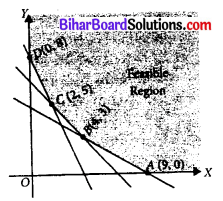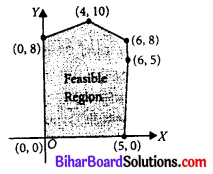# Bihar Board 12th Maths Objective Answers Chapter 12 Linear Programming

Bihar Board 12th Maths Objective Questions and Answers

## Bihar Board 12th Maths Objective Answers Chapter 12 Linear Programming

Question 1.
Objective function of a L.P.P.is
(a) a constant
(b) a function to be optimised
(c) a relation between the variables
(d) none of these
(b) a function to be optimised

Question 2.
The optimal value of the objective function is attained at the points
(a) on X-axis
(b) on Y-axis
(c) which are comer points of the feascible region
(d) none of these
(c) which are comer points of the feascible regionQuestion 3.
In solving the LPP:
“minimize f = 6x + 10y subject to constraints x ≥ 6, y ≥ 2, 2x + y ≥ 10, x ≥ 0, y ≥ 0” redundant constraints are
(a) x ≥ 6, y ≥ 2
(b) 2x + y ≥ 10, x ≥ 0, y ≥ 0
(c) x ≥ 6
(d) none of these
(b) 2x + y ≥ 10, x ≥ 0, y ≥ 0

Question 4.
Region represented by x ≥ 0, y ≥ 0 is

Question 5.
The region represented by the inequalities
x ≥ 6, y ≥ 2, 2x + y ≤ 0, x ≥ 0, y ≥ 0 is
(a) unbounded
(b) a polygon
(c) exterior of a triangle
(d) None of these
(d) None of theseQuestion 6.
Feasible region for an LPP is shown shaded in the following in the following figure. Minimum of Z = 4x + 3y occurs at the point(a) (0, 8)
(b) (2, 5)
(c) (4, 3)
(d) (9, 0)
(b) (2, 5)

Question 7.
Maximize Z = 3x + 5y, subject to x + 4y ≤ 24, 3x + y ≤ 21, x + y ≤ 9, x ≥ 0, y ≥ 0.
(a) 20 at (1, 0)
(b) 30 at (0, 6)
(c) 37 at (4, 5)
(d) 33 at (6, 3)
(c) 37 at (4, 5)

Question 8.
Maximize Z = 4x + 6y, subject to 3x + 2y ≤ 12, x + y ≥ 4, x, y ≥ 0.
(a) 16 at (4, 0)
(b) 24 at (0, 4)
(c) 24 at (6, 0)
(d) 36 at (0, 6)
(d) 36 at (0, 6)

Question 9.
Maximize Z = 6x + 4y, subject to x ≤ 2, x + y ≤ 3, -2x + y ≤ 1, x ≥ 0, y ≥ 0.
(a) 12 at (2, 0)
(b) $$\frac{140}{3}$$ at ($$\frac{2}{3}$$, $$\frac{1}{3}$$)
(c) 16 at (2, 1)
(d) 4 at (0, 1)
(c) 16 at (2, 1)

Question 10.
Maximize Z = 10×1 + 25×2, subject to 0 ≤ x1 ≤ 3, 0 ≤ x2 ≤ 3, x1 + x2 ≤ 5.
(a) 80 at (3, 2)
(b) 75 at (0, 3)
(c) 30 at (3, 0)
(d) 95 at (2, 3)
(d) 95 at (2, 3)Question 11.
Z = 20x1 + 20x2, subject to x1 ≥ 0, x2 ≥ 0, x1 + 2x2 ≥ 8, 3x1 + 2x2 ≥ 15, 5x1 + 2x2 ≥ 20. The minimum value of Z occurs at
(a) (8, 0)
(b) $$\left(\frac{5}{2}, \frac{15}{4}\right)$$
(c) $$\left(\frac{7}{2}, \frac{9}{4}\right)$$
(d) (0, 10)
(c) $$\left(\frac{7}{2}, \frac{9}{4}\right)$$

Question 12.
Z = 7x + y, subject to 5x + y ≥ 5, x + y ≥ 3, x ≥ 0, y ≥ 0. The minimum value of Z occurs at
(a) (3, 0)
(b) $$\left(\frac{1}{2}, \frac{5}{2}\right)$$
(c) (7, 0)
(d) (0, 5)
(d) (0, 5)

Question 13.
Minimize Z = 20x1 + 9x2, subject to x1 ≥ 0, x2 ≥ 0, 2x1 + 2x2 ≥ 36, 6x1 + x2 ≥ 60.
(a) 360 at (18, 0)
(b) 336 at (6, 4)
(c) 540 at (0, 60)
(d) 0 at (0, 0)
(b) 336 at (6, 4)

Question 14.
Z = 8x + 10y, subject to 2x + y ≥ 1, 2x + 3y ≥ 15, y ≥ 2, x ≥ 0, y ≥ 0. The minimum value of Z occurs at
(a) (4.5, 2)
(b) (1.5, 4)
(c) (0, 7)
(d) (7, 0)
(b) (1.5, 4)Question 15.
Z = 4x1 + 5x2, subject to 2x1 + x2 ≥ 7, 2x1 + 3x2 ≤ 15, x2 ≤ 3, x1, x2 ≥ 0. The minimum value of Z occurs at
(a) (3.5, 0)
(b) (3, 3)
(c) (7.5, 0)
(d) (2, 3)
(a) (3.5, 0)

Question 16.
The maximum value of f = 4x + 3y subject to constraints x ≥ 0, y ≥ 0, 2x + 3y ≤ 18; x + y ≥ 10 is
(a) 35
(b) 36
(c) 34
(d) none of these
(d) none of these

Question 17.
The minimum value of Z = 4x + 3y subjected to the constraints 3x + 2y ≥ 160, 5 + 2y ≥ 200, 2y ≥ 80; x, y ≥ 0 is
(a) 220
(b) 300
(c) 230
(d) none of these
(a) 220

Question 18.
The maximum value of Z = 3x + 2y, subjected to x + 2y ≤ 2, x + 2y ≥ 8; x, y ≥ 0 is
(a) 32
(b) 24
(c) 40
(d) none of these
(d) none of theseQuestion 19.
Maximize Z = 11x + 8y, subject to x ≤ 4, y ≤ 6, x ≥ 0, y ≥ 0.
(a) 44 at (4, 2)
(b) 60 at (4, 2)
(c) 62 at (4, 0)
(d) 48 at (4, 2)